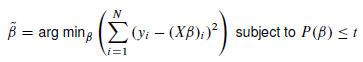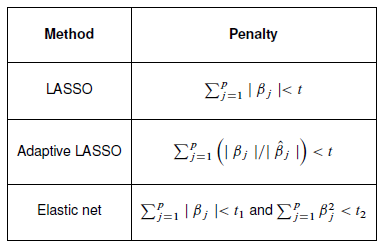## Tip: Top five reasons for using penalized regression for modeling your high-dimensional data

Started ‎04-09-2015 by
Modified ‎01-06-2016 by
Views 7,479

High-dimensional data are large and complex data in which the number of predictive variables can be anywhere from a few dozen to many thousands.  Applications are common in scientific fields such as genomics, tumor classifications, face recognition, and biomedical imaging.  Business problems with large numbers of predictors include scoring credit risk, predicting retail customer behavior, exploring health care alternatives, and tracking the effect of new pharmaceuticals in the population.

Penalized regression methods are modern regression methods for analyzing high-dimensional data. You can think of penalized regression methods as alternatives to traditional selection methods such as forward, backward and stepwise selection for fitting linear or logistic regression models.

This is my first post in a series of posts that will be collected under “Penalized Regression Tips”.  Here are my top five reasons why you should try penalized regression for your next predictive modeling and variable selection project. Penalized regression methods:

1. Provide interpretable models
2. Select variables and fit the model simultaneously – two birds with one stone!
3. Provide stable results and high prediction accuracy
4. Are computationally very efficient!
5. Do not require pre-training reduction!

Interpretability

Penalized regression methods fit linear or logistic regression models. While fitting models they can perform variable selection by placing at least one penalty on the size of the regression coefficients. For high-dimensional data, a linear model with carefully selected predictor variables works well because a linear model is easy to interpret and the small number of predictor variables enables you to understand the underlying processes that generate your data. To understand why interpretability is so important, think about the following example scenario: If you are a lender who uses a statistical model to screen customers’ applications, you need to not only make accurate predictions but also explain why an application was accepted or denied.

Simultaneous Model Fitting and Variable Selection

For regression problems with large number of predictor variables, traditional selection methods can be computationally very intense, because they first identify a subset of predictor variables by successively adding and/or removing variables, then use least squares estimation to fit a model based on the reduced set of predictors.  Penalized regression methods, on the other hand, select variables and fit models simultaneously.

Predictive Accuracy

For fitting linear models, traditional model selection methods achieve simplicity, but they have been shown to yield models that are often unstable and have low prediction accuracy, especially for high-dimensional data which often include many correlated predictor variables. For analyzing these type of data, penalized regression methods such as LASSO selection have become an attractive alternative to traditional selection because they can produce more stable models with higher prediction accuracy.

Computational Efficiency

The computational cost of fitting a penalized regression model can be less than one least squares estimation by using computationally efficient algorithms such as the LARS algorithm that solves LASSO.

No Pre-training Reduction Necessary

Most penalized regression methods can be directly applied to wide data where the number of predictors is much larger than the sample size.

Methods

Penalized regression for linear models solves the following constrained minimization problem:The following table shows popular penalized regression methods and their penalties (P(b)<t)):Penalized regression methods, LASSO, adaptive LASSO, and elastic net are available in the GLMSELECT procedure of SAS/STAT. You can also perform LASSO and adaptive LASSO by using the LARS node, HP Regression node, and the HP Variable Selection node of SAS Enterprise Miner™.

If these sound interesting to you, be sure to check out my upcoming posts about penalized regression. For more information about penalized regression, see the following papers and the short video:

Version history
Last update:
‎01-06-2016 01:44 PM
Updated by:Contributors
Article Labels
Article Tags